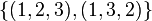# 3-cycle in symmetric group:S3

We consider the group$G$ defined as symmetric group:S3, i.e., the symmetric group of degree three, which for convenience we take to be the symmetric group acting on the set$\{ 1, 2, 3 \}$.

We are interested in the conjugacy class of 3-cycles in this group, i.e., permutations that move all elements and have order three. The 3-cycles form a single conjugacy class and also form a single orbit under the action of the automorphism group of$S_3$.

The full list of elements in the conjugacy class is:$\{ (1,2,3), (1,3,2) \}$

## Arithmetic functions

Function Value Explanation
order of the whole group 6
size of conjugacy class 2
size of automorphism class 2
number of conjugacy classes in automorphism class 1
order of elements in conjugacy class 3

## Description in alternative interpretations of the whole group

Interpretation of$G$ Description of conjugacy class Verification of size computation Verification of element order computation More information
As the symmetric group of degree three corresponds to the partition 3$\frac{3!}{3} = 2$$\operatorname{lcm}\{ 3 \} = 3$ element structure of symmetric groups, element structure of symmetric group:S3#Interpretation as symmetric group, conjugacy class size formula in symmetric group
As the dihedral group$D_{2n}$,$n = 3, 2n = 6$ ($n$ odd) the generator of the cyclic piece of order$n$ 2 (valid for$n \ge 3$)$n = 3$ element structure of dihedral groups, element structure of symmetric group:S3#Interpretation as dihedral group
As the special linear group$SL(2,q)$,$q = 2$ ($q$ even) the unique conjugacy class of semisimple elements not diagonalizable over the field itself$q(q - 1) = 2(2 - 1) = 2$ Must divide$q^2 - 1$ in general, in this case, it is$q^2 - 1 = 2^2 - 1 = 3$ element structure of special linear group of degree two over a finite field, element structure of symmetric group:S3#Interpretation as general linear group of degree two
As the general affine group$GA(1,q)$,$q = 3$ the conjugacy class of all maps of the form$x \mapsto x + b, b \in \mathbb{F}_3^\ast$.$q - 1 = 2$$q = 3$ element structure of general affine group of degree one over a finite field, element structure of symmetric group:S3#Interpretation as general affine group of degree one